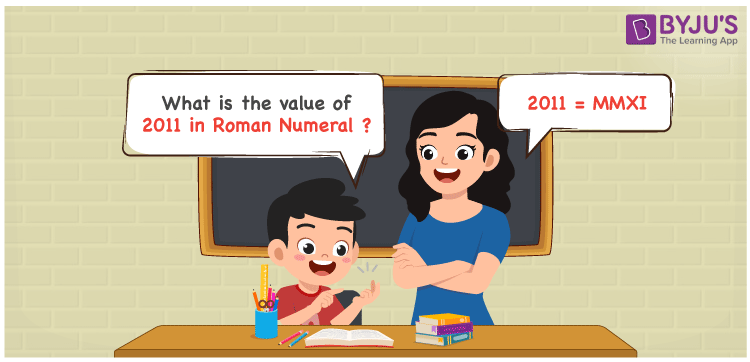# 2011 in Roman Numerals

2011 in Roman Numerals is MMXI. Any Roman numeral uses only the 7 primary symbols which are I, V, X, L, C, D, and M where M represents 1000, X represents 10 and I represents 1. MMXI follows the addition rule of Roman numerals and it is the number 2011. What are Roman numerals? can be referred for more details. The details on how to write 2011 is given in the next section of this article.

 Number Roman Numeral 2011 MMXI

## How to Write 2011 in Roman Numerals?To convert 2011 in Roman Numerals, we need to represent 2011 as the sum of the fundamental symbols. 2011 can be broken down as the sum of 1000,1000, 10 and 1. Since M is the symbol for 1000, 2000 will be MM, Similarly, 11 will be XI.

2011 = 1000 + 1000 + 10 + 1

2011 = M + M + X + I

2011 = MMXI.

## Video Lesson on Roman Numerals## Frequently Asked Questions on 2011 in Roman Numerals

Q1

### How to write the number 2011 in Roman Numerals?

2011 in Roman Numerals is written as MMXI.
Q2

### Does MMXI represent Roman Numerals?

Yes. MMXI represents Roman Numerals and it is the number 2011
Q3

### What is the year 2011 in Roman numerals?

The year 2011 in Roman Numerals is MMXI.